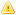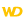This content has been translated automatically.  Click here  to view the French version.
• Overview
• Detail
• Flexible equality and very flexible equality
• Equality and comparison: Real containing more than 6 different decimal places
• Comparison intervals
• Comparison operators and UNICODE
• Comparing instances of structures and instances of classes
• IN operator
WINDEVWEBDEVWINDEV MobileOthersComparison operators
Overview
The comparison operators can be divided into several categories:
 Equality Strict equality: =Flexible equality: ~=Very flexible equality: ~~ Starts with: [= Comparison Different: <>Less than or equal to: <=Greater than or equal to: >=Strictly less than: Starts with: [=Starts with: [~ (ignores the space characters found at the beginning of string and the case).Starts with: [~~ (ignores all the space characters, the punctuation and the case).Comparison to a list of values: INContains: [=]Contains: [~] (ignores the case and the space characters found at the beginning and end of string).Contains: [~~] (ignores all the space characters, the punctuation and the case)Ends with: =]Ends with: ~] (ignore the space characters at the end of string and the case)Ends with: ~~] (ignore the space characters, the punctuation and the case) Comparison interval Strictly included between: < .. < (and conversely)Value1 < x < Value2Between: < .. <= AND < .. = < (and conversely)Value1 <= x AND x <= Value2Included between (including bounds): <= <= (and conversely)Value1 <= x <= Value2Included between (including bounds): x TO y
The comparison operators can be used with all the types of operands.
The result of a comparison expression is a boolean.
Detail

Flexible equality and very flexible equality

The flexible equality (~=) only applies to the character strings (except the fixed strings). This operator allows you to:
• make no difference between the uppercase characters and the lowercase characters,
• ignore the space characters found before and after the string whose test must be run,
• ignore the lowercase accented characters.
To ignore the space characters, the punctuation characters and the CR characters inside the strings, use the very flexible equality (~~).
HFSQL equivalence: To retrieve the equivalence to the very flexible equality when performing a search on a text key in an HFSQL data file, the following options must be configured when the item is described in the analysis:The comparison takes into account the accented lowercase characters in Browser code.The "Very flexible equality" operator is not available in browser code.
"Dupond" = "DUPOND"                // Retourne Faux
"Dupond" ~= "DUPOND"                // Retourne Vrai
" Dupond" ~= "DUPOND"               // Retourne Vrai
" Dupond" ~= "Dupond"              // Retourne Vrai
"C'est l'été" ~= "C'est l'ete"     // Retourne Vrai
"Ent. S.A.R.L" ~~ "Ent SARL"        // Retourne Vrai

Equality and comparison: Real containing more than 6 different decimal places

The test of equality between two real numbers is performed according to the first 6 decimal places. Indeed, the rounding errors caused by the internal coding of the reals require a specific test.
This test must be run by comparing the difference between the two values to test and a reference value. Depending on the type of your application, this value can be equal to 0.00001 or even less.
This rounding management is not specific to WINDEV, WEBDEV and WINDEV Mobile. It is common to all the programming languages that handle the reals in binary format.
Diff, R1, R2 are reals
Diff = 0.00001
IF Abs(1-(R1/R2)) < Diff THEN
// R1 et R2 sont égaux
ELSE
// R1 et R2 sont différents
END

Comparison intervals

The comparison intervals are used to simplify the syntax of complex comparisons.
Some examples of identical comparisons:
• Example 1:
• IF x>5 AND x<10 THEN...
• IF 5<x<10 THEN...
• Example 2:
• IF x>=5 AND x<=10 THEN...
• IF x=5 TO 10 THEN ...Not supported.
MonTableau is array of 5 strings
I is int
MonTableau = "Dupond"
MonTableau = "Aida"
MonTableau = "Parapoline"
MonTableau = "Moulin"
MonTableau = "Foolamour"
FOR I = 1 TO 5
IF "B" < MonTableau[I] <= "M" THEN Trace(MonTableau[I])
// Affiche Dupond et Foolamour
END

Comparison operators and UNICODE

The available operators are as follows:
• "=": Strict equality
• "<>": Difference
• "[=": Starts with
• "[~": Starts with while ignoring the space characters found at the beginning of the string and while ignoring the case.
• "[~~": Starts with while ignoring the space characters, the punctuation characters and the case.
• Contains: [=]
• Contains: [~]: Contains while ignoring the case.
• Contains: [~~]: Contains while ignoring all the space characters, the punctuation characters and the case.
• Ends with: =]
• Ends with: ~]: Ends with while ignoring the space characters found at the end of the string and while ignoring the case.
• Ends with: ~~]: Ends with while ignoring the space characters, the punctuation and the case.
• "<", "<=", ">=", ">": Strictly less than, less than or equal to, greater than or equal to, strictly greater than
You have the ability to use ANSI strings, Unicode strings and buffers in the different parameters of the comparison.
The following conversion rule is used for the Ansi systems (Windows or Linux):
• If at least one of the strings is a buffer, all the strings are converted to buffers and the operation is performed with buffers,
• If the first condition is not met and there is at least one Unicode string, all the strings are converted to Unicode and the operation is performed in Unicode (the conversion is performed with the current character set, if necessary),
• Otherwise, the operation is performed in Ansi.
The following conversion rule is used for the Unicode systems (Windows CE):
• If at least one of the strings is a buffer, all the strings are converted to buffers and the operation is performed with buffers,
• Otherwise, the operation is performed in Unicode.
For more details on Unicode, see WINDEV and the Unicode format.Comparing instances of structures and instances of classes

The dynamic structures are instantiated when they are allocated.
You have the ability to compare instances of dynamic structures (or instances of dynamic classes) via the "=" operator.
Example:
// Déclaration de structures
O is ST1
P is ST2

// Déclaration et instanciation de structures dynamiques
P1 is Structure ST1 dynamic
P1 = O
P2 is Structure ST2 dynamic
P2 = O

// Comparaison
IF P1 = P2 THEN ...
The same operation can be performed on the instances of classes in order to find out whether two variables designate a single class instance.
The comparison between two different instances of a class can be performed by using buffers.
Example:
Objet1 is Classe1 dynamic
Objet2 is Classe1 dynamic
BufferObjet1, BufferObjet2 are Buffers

Objet1 = new Classe1
Objet2 = new Classe1

Serialize(Objet1, BufferObjet1, psdBinary)
Serialize(Objet2, BufferObjet2, psdBinary)

IF BufferObjet1 = BufferObjet2 THEN
// Vrai si les deux instances ont des contenus identiques
ELSE
// Faux si au moins un membre diffère
END

IN operator

The IN operator is used to compare a value to a list of values. Its evaluation returns a boolean.
Example:
MaValeur is int
MonRésultat is boolean
MaValeur = 10
MonRésultat = (MaValeur IN(1,4,6,10))
The list of values can contain literal values or variables whose value will be evaluated before the comparison is performed.
Example:
MaValeur is int
MonAutreValeur is int
MonRésultat is boolean
MaValeur = 10
MonAutreValeur = 15
MonRésultat = (MaValeur IN(1,4,6,10,MonAutreValeur))This feature is not available.
Minimum version required
• Version 9